# Opinion of Prof. Vadim Utkin

## View of Old-Timer.

Concepts “Variable Structure Systems” and “Sliding Mode Control” were initiated half a century ago and now many researchers of different countries are working in this area enthusiastically. It is important to establish bridges between the original “old” ideas and new promising directions of last two decades to avoid misleading statements and exaggerated optimism.

### 1. About Relative Degree (RD)

I start with a primitive question related to the first order system, assuming that upper or low bounds of unknown disturbance F(t) (or its time derivatives) are known only. What is RD of the first order system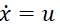(Fig.1)? Answer is evident RD=1. Now add an integrator in the input. Of course RD of the system with input v depends on the selected output variable: if x, then RD=2, if s, then RD=1.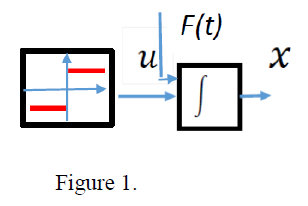The core condition: sliding mode can be enforced if and only if time derivative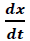is available. Therefore the system in Fig.2 is called system with RD=1 for more than 60 years.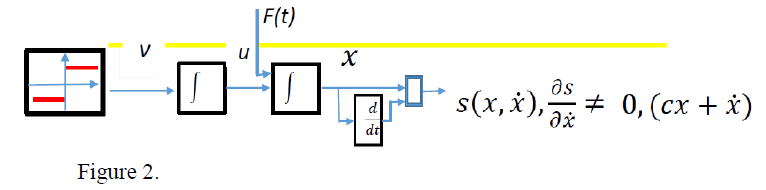The authors of publication of 60’s understood that RD=n for the system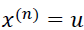and sliding mode cannot be enforced for control as a function output x. For its existence RD=1 is needed, therefore the output was differentiated (n-1) times. Similarly to our example, the core condition becomes: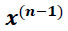should be available and for several decades nobody said, that the system with RD=n is controlled in sliding mode.

Unfortunately the similar statement is made in publications on high order sliding mode control (HOSM). The authors do exactly the same (as in publications of 60’s) for system with RD=k>1 between u and s (Fig.3). Output s is handled as x in the above examples. Then it is differentiated k-1 times to make RD=1 and sliding mode is enforced on the preselected manifold in the system state space. All actions are identical to the “old” methodology except for the final statement: sliding mode exists in the system with RD= k>1. I can accept neither the statement nor its interpretation as a new result. Otherwise we should admit that this “new result” was obtained 60 years ago.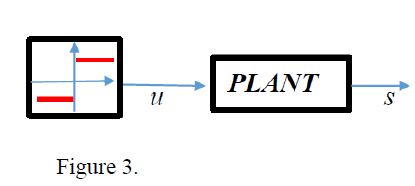Of course it is not criticism of HOSM as a new phenomenon. Manifold s of dimension n-k,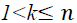is reached after a finite time interval (as for “old” sliding mode control with n-1-dimensional sliding surface) and then new type of sliding mode occurs, called HOSM of order k (of course in the system with RD=1).### 2. Sliding mode with continuous control

Again discuss this promising statement using systems in Fig.1 and Fig.2. Input v is discontinuous, intermediate variable u is continuous. Based on this fact the “new result” is announced in publications on HOSM: sliding mode can be implemented by continuous control. Objection is evident: sliding mode exists only if input v is discontinuous. Shorter: no discontinuity – no sliding mode. Otherwise this “new result” can be discovered easily in any sliding mode control system with a dynamic actuator, since its output is continuous (Fig.4).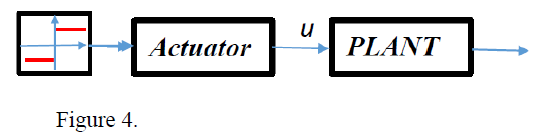Comment to “no discontinuity – no sliding mode”. Of course the special case should be
mentioned, when sliding mode can be enforced by a continuous non-Lipschitzian function. If
control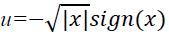in the first order system (Fig.1) with disturbance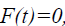, then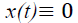after a finite time interval. However the control is equal to zero identically as well
and unable to reject the disturbances from the class indicated in Section 1.### 3.Rejection of unmatched disturbances

Again a promising and at the same time strange statement, because the matching condition is necessary and sufficient for an arbitrary disturbance rejection. Nevertheless the statement can be found in recent publication on HOSM. For example the state cannot be reduced to zero in the control system with unmatched arbitrary disturbance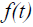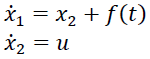The trick of the authors of these publications is evident. State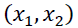is replaced by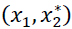,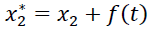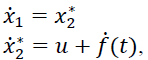where disturbance becomes matched and then the trivial problem is solved.

If we admit the trick as a “new result”, we should state that, generally speaking, the problem of unmatched disturbance does not exist. Indeed, any disturbance becomes matched in the state consisting of an output and its derivatives, as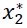is time derivative of output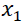in our second order system.### 4. Finite time convergence

4.1.HOSM was announced as an efficient tool for chattering suppression, for example chattering caused by unmodeled dynamics. But at the presence of chattering finite time convergence is not of any value. Convergence to what?

4.2. HOSM implies finite time convergence to manifold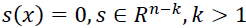, and then sliding mode in this manifold. But if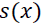is Lipschitzian function, then convergence in sliding mode is asymptotic (not finite time) and the total process is converging asymptotically. Then finite time of the first stage (reaching sliding manifold) is not of any value: the processes in HOSM systems are not converging in finite time, but asymptotically only. Then the processes with the same rate of convergence can be easily implemented by “old” sliding mode control. But “old” control is much simpler than HOSM control. Please compare two control functions of the same arguments resulting in the same processes for the case k=3

“Old” control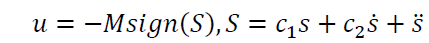HOSM control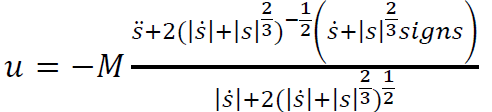The case n=3, k=2 is shown in Fig. 5 with green (“old” control) and red (HOSM control)
trajectories.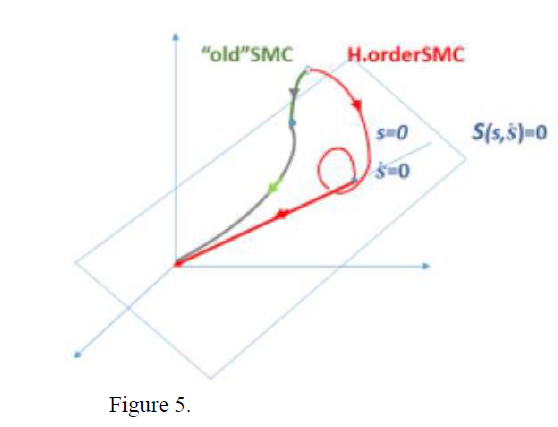### 5. Finite time convergence and chattering.

As stated in the previous section “old” and HOSM controls have similar processes, but implementation of the first one is much simpler. These two approaches cannot be compared when functionin HOSM control is not Lipschitzian and the origin in the state space is reached after a finite time. Chattering is the main obstacle for implementation of sliding mode control and it is caused by unmodeled dynamics along with discontinuous or high gain feedback. To have finite time convergence in sliding mode functiondoes not have Lipschitz constant in the origin and the local gain tends to infinity (Fig.6).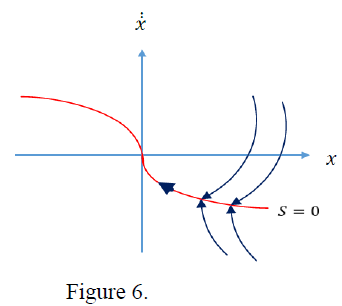It can result in higher level of chattering if compared with systems with asymptotic convergence. The simulation results confirmed this concern for the system in Fig.7 with asymptotic convergence,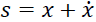(Fig.8a) and finite time convergence,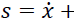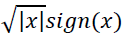(Fig.8b) after unmodeled dynamics with small time constant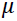were added. As can be seen the transient processes in both cases are similar, but level of chattering is more than five times higher for HOSM control.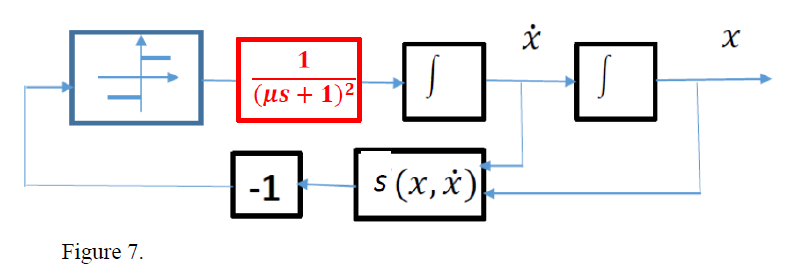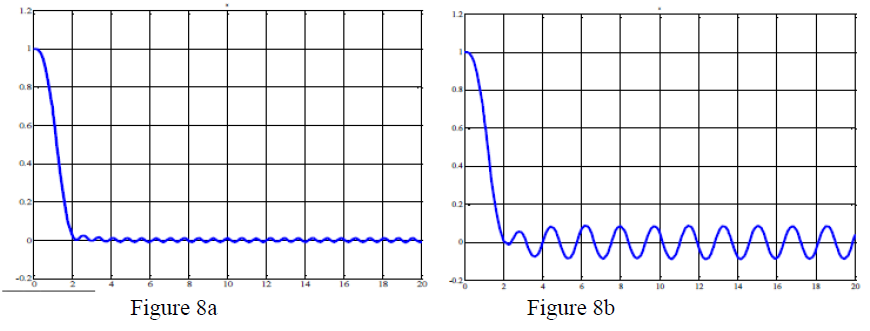Finite time convergence is in a conflict with chattering suppression problem.

In conclusion: the comments reflect only my view of the current tendencies in our area and
I would be happy to discuss them in any format.